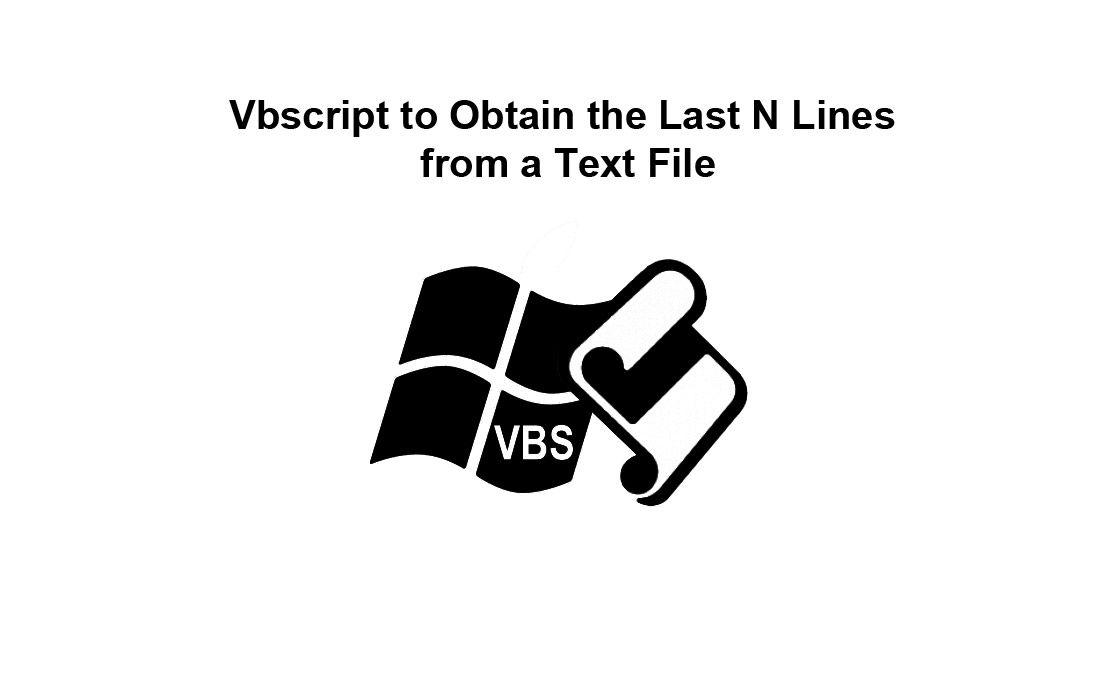# Vbscript to Obtain the Last N Lines from a Text FileTail.vbs borrows shamelessy from unix’ tail command, and more or less performs the same function: to obtain the last N lines of a textfile (the tail).

Usage 1 (content is obtained from the filename given on the commandline):
[cscript] TAIL[.vbs] path+filename startline [numberlines]
where ‘startline’ is counted from the end of the file, and numberlines is how many lines to obtain after that  point, and defaults to “through end-of-file”
If your filename happens to be a number, be sure to include a full or relative path in front of it so it will be interpreted as a filename. F/e, if you have a file called 6, or 3.2, refer to it like: .\3.2 or c:\x\6

Usage 2 (content is obtained through the pipe)
… | CSCRIPT TAIL.VBS startline [numberlines]
Same idea, but CSCRIPT must be used to launch the vbs, it will not run implicitly.

testfile:
3 from end
2 from end
1 from end

examples:
tail testfile 3 1 == 3 from end
tail testfile 3   == 3 from end [crlf]2 from end[crlf]1 from end
tail testfile 1   == 1 from end
type testfile | cscript tail.vbs 3 1 == 3 from end

`'======== begin vbscript "tail"  `
`set fso=createobject("scripting.filesystemobject")  `
`argcnt=wscript.arguments.count  `
`if argcnt>0 then file=wscript.arguments(0)  `
`if argcnt>1 then tail=wscript.arguments(1)+1  `
`tailend=0  `
`select case argcnt  `
`case 1  `
`'one arg, only interp as line number, use stdin as file  `
` if not isnumeric(file) then wscript.quit  `
` b=""  `
` on error resume next  `
` b=wscript.stdin.readall  `
` if b="" then wscript.quit  `
` tail=file+1  `
`case 2  `
`'two args: file and line, or two lines and stdin for file  `
` if isnumeric(file) then 'two numbers  `
`  b=""  `
`  on error resume next  `
`  b=wscript.stdin.readall  `
`  if b="" then wscript.quit  `
`  tail=file+1  `
`  tailend=tail-wscript.arguments(1)  `
` else  `
`  set a=fso.opentextfile(file,1) 'non-number, use as file  `
`  b=a.readall  `
` end if  `
`case 3  `
`'easiest: file, line1, line2  `
` tailend=tail-wscript.arguments(2)  `
` set a=fso.opentextfile(file,1)  `
` b=a.readall  `
`end select  `
`p=-1  `
`for i=1 to tail  `
`if i=tailend+1 then p2=p  `
`p=instrrev(b,vbcrlf,p)  `
`if p=0 then  `
` p=1  `
` exit for  `
`end if  `
`next  `
`if p2>0 then c=mid(b,p,p2-p) else c=mid(b,p)  `
`wscript.echo mid(c,3)  `
`'======= end vbscript`